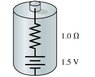# Find the value of the emf in a battery

• rayhan619
In summary, a real battery can be modeled as a 1.5 emf in series with a resistor, known as the internal resistance. When there is no current flowing through the battery, the potential difference between its terminals is 1.5 V. However, when connected to a 3.0 Ohm resistor, the voltage difference is 4.5 V. This means that the internal resistance dissipates a fraction of the battery's power, which can be calculated using V=IR.

## Homework Statement

A real battery is not just an emf. We can model a real 1.5 battery as a 1.5 emf in series with a resistor known as the "internal resistance", as shown in the figure (Intro 1 figure) . A typical battery has 1.0 Ohm internal resistance due to imperfections that limit current through the battery. When there's no current through the battery, and thus no voltage drop across the internal resistance, the potential difference between its terminals is 1.5 V, the value of the emf. Suppose the terminals of this battery are connected to a 3.0 Ohm resistor.
a) What is the potential difference between the terminals of the battery?

b) What fraction of the battery's power is dissipated by the internal resistance?

V = IR

## The Attempt at a Solution

here is the pic

#### Attachments

•1.jpg
7.4 KB · Views: 840

Yes you do need V=IR but show your attempt to use it.

V = IR
I = V/R = 1.5 V/1 Ohm = 1.5 A

so for 3 Ohm resistor voltage difference, V = IR = 1.5 A*3 Ohm = 4.5 V

Right?

I=V/R but when the resistor is connected the total resistance=4 ohms.The same current flows through The internal resistance of the battery and the 3 ohm resistor.From this you should be able to work out part a which can be looked at in two ways.
1.The voltage across the 3 ohm resistor
2.The emf minus the voltage dropped across the internal resistance
Sketch the circuit,mark in the voltages and their polarities and you should see why the two answers are the same.
Try it out and show what you get.

so the resistance is 4 ohm and the voltage is 1.5 V
so I = V/R = 1.5/40 = 0.375 A
right?

Right, now you should be able to finish it off.